• Category
• >Machine Learning

# Introduction to Model Hyperparameter and Tuning in Machine Learning

• Rohit Dwivedi
• May 23, 2020
• Updated on: Jan 18, 2021Model Hyperparameters are the assets that take care of the whole training of an algorithm. While an algorithm learns the model parameter from the data, the hyperparameters are used to power the behavior of the algorithm. These parameters are initialized before any training of the algorithm takes place. Let us see the differences between model parameters and hyperparameters.

## Model Parameters vs Hyperparameters

Model parameters are about the weights and coefficient that is grasped from the data by the algorithm. Model parameters contemplate how the target variable is depending upon the predictor variable. Hyperparameters solely depend upon the conduct of the algorithms when it is in the learning phase. Every algorithm has its clear-cut set of hyperparameters like for decision trees it is a depth parameter

The hyperparameter is the default parameter that works in all situations. They are termed as an important part of a model. It is not necessary that you can only use the default parameters you can make tweaks if the situation demands. It is important to have three sets in which data is divided like training, testing, validation set whenever you make tweaks in the default parameter to get the necessary accuracy so as to stop data leaks.

## Regularising Linear Models (Shrinkage methods)

When there are many parameters and are uncovered to the imprecation of dimensionality, there is a need to center to dimensionality techniques like principal component analysis and removing the PCA with the slightest magnitude of eigenvalues. Before it is found out the correct number of principal components, this process can be difficult. Alternatively, shrinkage methods can be implemented. This method diminishes the coefficient and results in simple and also effective models. The two shrinkage methods are discussed below:

### Ridge Regression

It is similar to linear regression where the aim is to get the best fit surface. The difference that makes each other different is the method of finding the best coefficients. In the case of ridge regression optimization function different from the SSE that is used in linear regression.

Y1 = a0 + a1X + ε

linear regression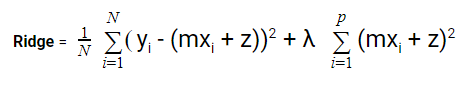Ridge Regression function

𝝺 is used as the penalty term used to penalize the bigger enormity coefficients, these are repressed significantly. The cost function becomes 0 when the value is assigned as 0 which is similar to the linear regression cost function.

### Why should you shrink the coefficients?

It is pleasant to shrink the coefficients as the models become composite, overfit, and liable to variance errors when there are a large number of dimensions and only a few data points. When you will check about the coefficients of the features of these composite models, you will get to know that the enormity of different coefficients become big. This specifies if you will make a unit change in the input variable, the change in the magnitude of the target column would be very large.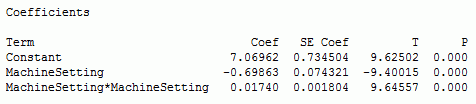Coefficients of a model

The algorithm that tries to search out the best combination of coefficients in ridge regression, that aims to reduce the SSE on training data, is mannered by penalty term. The penalty term is similar to the cost of the magnitude of the coefficients, if the enormity is high, more would be the cost. Therefore, the coefficients are repressed to lower the cost. Consequently, the resulting surface inclines to be more plane than the spontaneous surface that means the model would make an error in the training data. This can be okay until the eros can be featured to the random variation. These models would be efficiently well on test data. It would be able to generalize in a better way with respect to complex models.

### Lasso Regression

• It is similar to the Ridge regression, the only difference is the penalty term. The penalty term in lasso is raised to power 1. It is also called the L1 norm.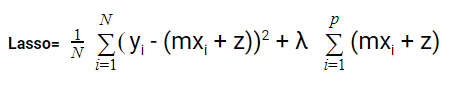Lasso Function

• As the input parameter the term resume that decides how big penalties would be for the coefficients. If high is the value more shrink the coefficient would be.

•

In Ridge regression, the coefficients are navigated towards zero but might not be zero in the lasso regression penalty process would make many of the coefficients 0, or it removes the dimensions

``````from sklearn import linear_model
reg = linear_model.Lasso(alpha=0.1)
reg.fit([[0, 0], [1, 1]], [0, 1])
``````

>>> Lasso(alpha=0.1)

``reg.predict([[1, 1]])``

>>> array([0.8])

Impact of Lasso Regression on the coefficients

Bigger coefficients have been repressed to 0, removing those dimensions. Check the documentation of ridge there on sklearn here and lasso here.

## Hyper Parameters & Tuning

• Hyperparameters control the behavior of the algorithm that is used for modeling.

• Hyperparameters are passed in the arguments during the initialization of the algorithm. (Ex. Specifying the criterion for decision tree building)

• If you want to check about the hyperparameters for an algorithm you can make use of the function get_params().

• Suppose you want to get the hyper parameter of SVM Classifier. 1) from sklearn.svm import SVC 2) svc = SVC() 3) svc.get_params()

• ### Fine Tuning the Hyper Parameters

1. Selection of the type of model whether classifier or regressor.
2. Look for the parameter space.
3. Choose the way for sampling parameter space.
4. Use the cross-validation scheme to make sure the model will be able to generalize.
5. Choose the function for scoring so as to validate the model.

• ### Approach to search for hyper parameter space

1. GridSearchCV - It takes all the parameters combination.
2. RandomizedSeachCV - It can sample a given number of possibilities with certain distribution from a parameter space.

• During tuning of the hyper parameters the data should always be divided into three parts that are training, validation, and testing so as to stop data leak.

• The same set of functions should be used to transform the test data separately that were used to transform the rest of the data for building models and doing hyperparameter tuning.

### GridSearchCV

• It is known as the hyperparameter tuning method.

• For all the given hyperparameter values GridSearchCV builds a model for every permutation.

• Every model that is built is validated and ranked.

• The best performing model having the best hyperparameters values is taken.

• For each permutation, cross-validation is used for evaluation, and scores are calculated.

• This is a comprehensive sampling of the hyperparameter space and can be ineffectual.

Check the documentation of GridSearchCV present in the sklearn library here.

### RandomizedSeachCV

• Random search is different from the grid search. Statistical distribution is provided instead of giving a separate set of values to inspect on each hyperparameter.

•  The combined distribution values of the different hyperparameters are picked up randomly.

• The reason for using random search instead of grid search is because in many instances hyperparameters are not fairly important.

• Not every combination is evaluated with respect to GridSearchCV. The fixed no of parameter settings is selected from the respective distribution.

• N_iter is used to denote the tried parameters.

• Random Search has more chance of getting the correct combination than the grid-search.

Check the documentation of RandomizedSearchCV present in the sklearn library here.

## What is a pipeline?

• There are situations where we need to tag all the different processes that were done to prepare the data for the machine learning model.

• Pipeline class that is present in sklearn helps you build a single process that consists of different processes that were done. It contains every process that was done while preparing the data for the model.

• Pipeline when used with GridSearchCV will lead to having findings over the hyperparameter space i.e at every stage.

• Initially in the pipeline, the list of transforms is put in and the final estimator.

• The next steps of the pipeline should be ‘transforms’, that is, implementing a fit & transform method.

• The final approximation only needs to instrument fit.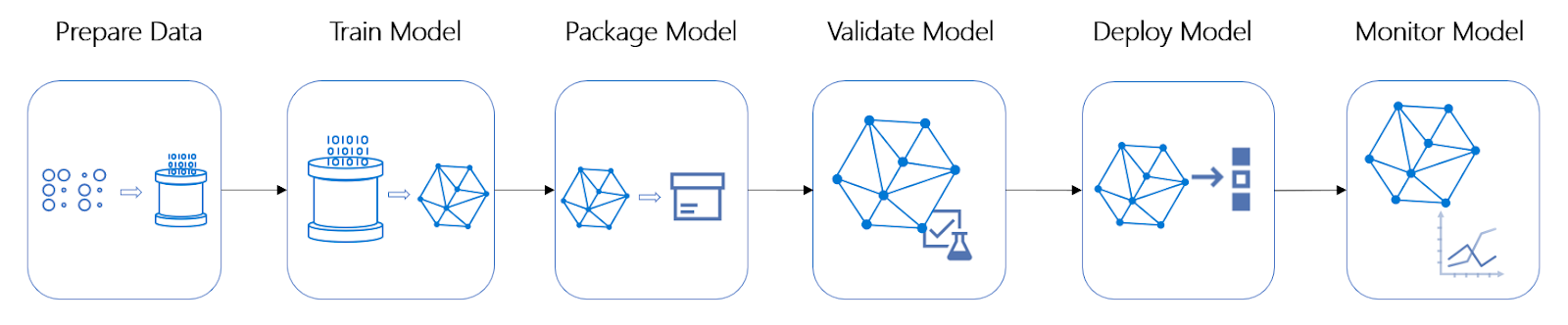Pipelining, source

### How to build a pipeline?

You need to first import the pipeline class that can be done using the syntax, “from sklearn.pipeline import Pipeline

• Initiate the object of the class by listing out the transformation steps.

“ Pipe = Pipeline([(“scale”,MinMaxScaler()),(“svm”,SVC())]) ”.

• Next step is to call the fit() function on the pipeline object.

“pipe.fit( X_train, y_train)”.

• Final steps are to call the score() function on the pipeline object or predict() function.

“pipe.score(X_test,y_test)”

“pipe.predict(X_test,y_test)”

## What is Sampling?

• There is a need for the right features, true class representation for the sample to be near characteristics of the population.

• The problem comes when there is a classification task and data is not balanced that means classes are imbalanced.

• The ML model can give negative results when there are imbalanced classes.

• Algorithms like Decision Tree, Logistic Regression were designed to lower down the overall inaccuracies, therefore gets biased towards over illustrative class.

• When class of significance is under represented, there can be no amount of tuning the model that could help.

• Consider a case where in data there defaulters are only 4 out of a total 100 individuals and there the class of importance is the defaulters class. Conventional classifiers will be prone to have high rate of type II errors that defaulters predicted as non defaulters.

### How to handle the Class Imbalance?

• To handle the imbalance data and to lower down the type II errors there can be balancing of the class representations.

• This can be done in two ways:
• To increase the samples of minority class.
• To decrease the sample of majority class.
• To increase the samples of minority class random oversampling is used.
• Total samples - 1000

• Defaulters - 20

• Non-defaulters - 980

• Event rate of interest- 2%

• Replicate a % of default cases n times for example, 10 cases 20 times

• Observation increased from 1000 to 1200

• Updated event rate -  220/1200  = 18%

• To decrease the samples of majority class random under sampling is used.

• Total samples - 1000

• Defaulters - 20

• Non-defaulters - 980

• Event rate of interest- 2%

• Pick 10% of non-defaulters cases randomly -  98

• Mix with defaulters cases - 118 observations.

• Updated event rate -  22/118  = 17%

• A simple way of doing over-sampling is to create random identical records from the minority class, that results in overfitting.

• In under-sampling, the easiest technique involves deleting random records from the majority class that would result in the loss of information.

### What are Imblearn techniques?

• There are more resampling techniques that are present in python’s imbalanced-learn module.

• There can clustering of records of the majority class and then undersampling can be done by deleting the records from every cluster, therefore conserving the information.

• In the case of over-sampling, rather than replicating the minority class records, there can be introduction of small changes into those replications that would result in more diverse synthetic samples.

• #### Synthetic Minority oversampling technique (SMOTE)

1. Based on the elements that are already present it consists of synthesizing elements.
2. It works by computing KNN for the randomly chosen point from the minority class.
3. Between the chosen point and its neighbors, synthetic points are added.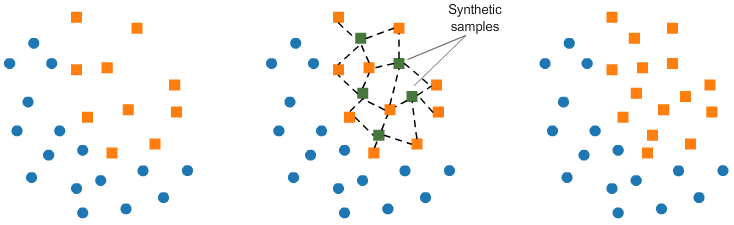SMOTE, source

1. These are the pairs of opposite classes having close instances.
2. When the majority class instances are removed there gets to expand the space between both the classes that facilitate the classification process.• #### Cluster centroid based under sampling

1. It uses the K-means algorithm to replace the cluster of majority observations to under sample the majority class.

2. By fitting the K-means algorithms it keeps the N majority samples with N cluster to the majority class and uses the coordinates of the N cluster centroids as the updated majority samples.

You can check the documentation of imbalanced-learn here and Github here. Also, you can check the combination of over and under sampling algorithms.

## Conclusion

I would conclude the blog by stating that hyperparameters are fundamental to the machine learning model. It can help you achieve reliable results. So in this blog, I have discussed the difference between model parameter and hyper parameter and also seen how to regularise linear models.

I have tried to introduce you to techniques for searching optimal hyper parameters that are GridSearchCV and RandomizedSearchCV. Also, I have explained the pipeline concept and how to build a pipeline. In the last section of the blog, I have introduced you to the sampling, how to handle the class imbalance and different imblearn techniques.